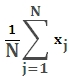# SQL Server average (arithmetic mean) function

Updated: 6 August 2010

Use AVERAGE_q to return the average (arithmetic mean) for a dataset. The equation for AVERAGE_q is:Syntax
SELECT [wctStatistics].[wct].[AVERAGE_q] (
<@Values_RangeQuery, nvarchar(4000),>)
Arguments
@Values_RangeQuery
the select statement, as text, used to determine the values to be used in the AVERAGE_q calculation.
Return Types
float
Remarks
·         AVERAGE_q is the arithmetic mean and is calculated by adding a group of numbers and then dividing by the count of those numbers. For purposes of this calculation, NULL values are not included.
·         No GROUP BY is required for this function even though it produces aggregated results.
·         AVERAGE_q may be used on normalized and de-normalized table, but for simpler queries, consider using AVERAGE
Examples

Using the normalized table #s1, calculate the average student grade for the Math.

SELECT round(wct.AVERAGE_q('Select Grade from #s1 where subject = ' + char(39) + 'Math' + Char(39)), 3)

This produces the following result

----------------------
87.822

(1 row(s) affected)

Using the normalized table #s1, calculate the average grade for each subject

SELECT distinct s2.subject
,Round(wct.AVERAGE_q('Select s1.Grade from #s1 s1 where s1.subject = ' + Char(39) + s2.subject + Char(39)), 3)
from #s1 s2

This produces the following result

subject
-------------------------------------------------- ----------------------
Foreign Language                                   84.897
History                                            91.72
Literature                                         91.72
Math                                               87.822
Science                                            89.77

(5 row(s) affected)

Using the normalized table #s1, calculate the average grade for each student

SELECT distinct s2.student
,Round(wct.AVERAGE_q('Select s1.Grade from #s1 s1 where s1.student = ' + Char(39) + s2.student + Char(39)), 3)
from #s1 s2

This produces the following result

student
-------------------------------------------------- ----------------------
Student 01                                         97.506
Student 02                                         94.734
Student 03                                         93.59
Student 04                                         92.776
Student 05                                         92.114
Student 06                                         91.542
Student 07                                         91.028
Student 08                                         90.554
Student 09                                         90.104
Student 10                                         89.672
Student 11                                         89.25
Student 12                                         88.83
Student 13                                         88.414
Student 14                                         87.986
Student 15                                         87.55
Student 16                                         87.092
Student 17                                         86.604
Student 18                                         86.076
Student 19                                         85.478
Student 20                                         84.776
Student 21                                         83.882
Student 22                                         82.526

(22 row(s) affected)

### SupportCopyright 2008-2023 Westclintech LLC         Privacy Policy        Terms of Service